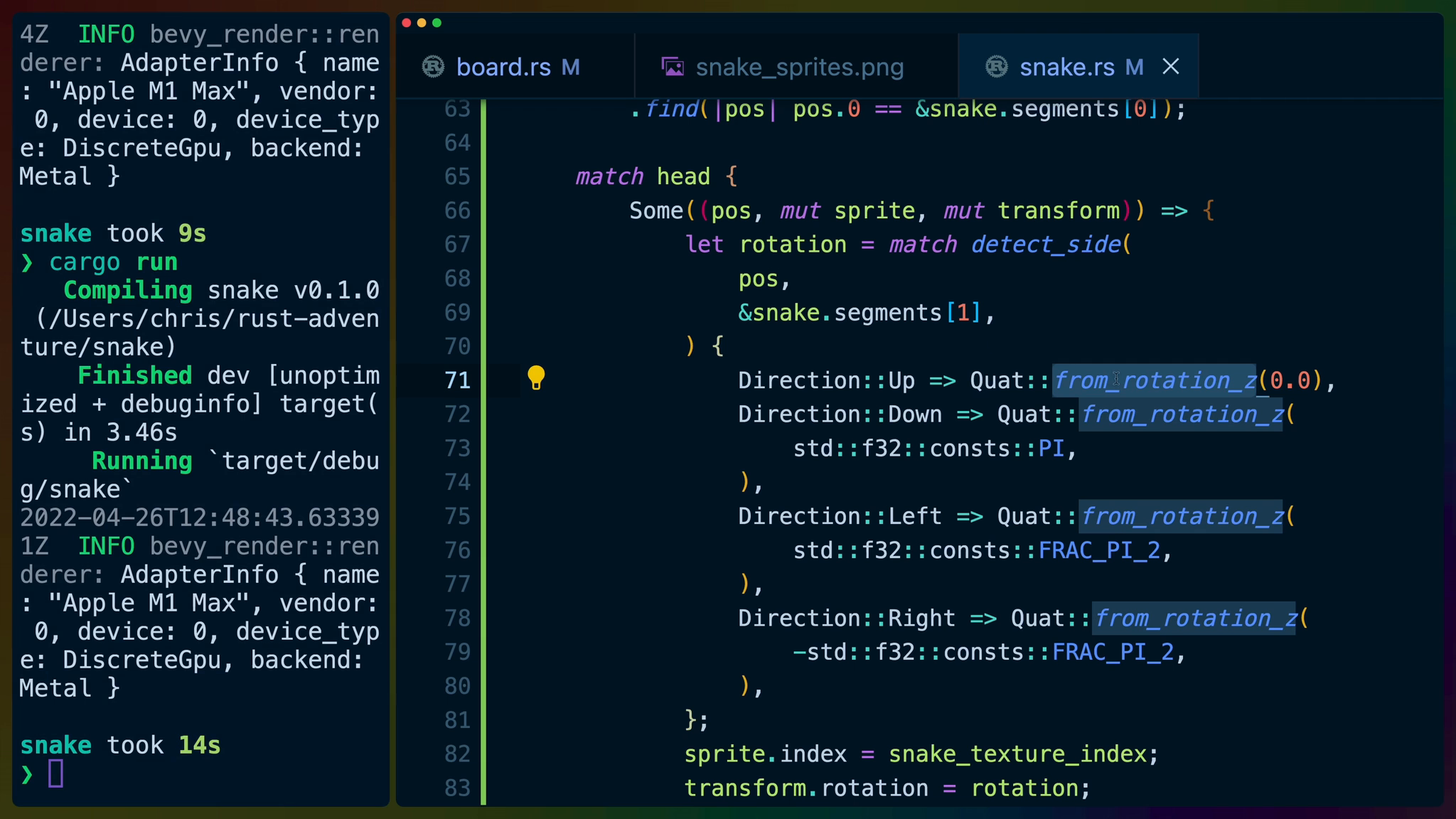### Lesson Details

The `snake_sprites` image from our assets looks something like this. It’s a 4x30 image containing the head, body, right angle, and tail for 30 snake variants.
In `board.rs` in our `SpawnSnakeSegment` command, we need to add some code to grab the snake texture from the assets resource.

``````let snake = world
.get_resource::<ImageAssets>()
.unwrap()
.snake
.clone();
``````

We also need to update our `SpriteBundle` to a `SpriteSheetBundle`, exactly like we did for the grass.

``````world
.spawn()
.insert_bundle(SpriteSheetBundle {
texture_atlas: snake,
sprite: TextureAtlasSprite {
index: 0,
custom_size: Some(Vec2::new(
TILE_SIZE, TILE_SIZE,
)),
..TextureAtlasSprite::default()
},
transform: Transform::from_xyz(x, y, 2.0),
..Default::default()
})
.insert(self.position);
``````

With an index of 0, we’ll see whale heads as our snake
If we change the index to 8, we get the snake, the third option. This is because the indexes in our `TextureAtlas` start at the top left, move right across the row and then move down to the next row. This means that because we have 4 columns that every snake head is going to be a multiple of 4: 0,4,8,12,etc.
That’s a lot of heads. We also need to work in the body (straight and right-angle), and the tail.

To pick the right sprite, we need to know its position in the VecDeque, and what sides the segments before and after exist on.

For example, a 3 segment snake in a vertical like moving upward would be: head, straight, tail.
A 3 segment snake going horizontally would also be head, straight, tail, but with a rotation of 90 degrees.
To start off, in `snake.rs` we’ll write a helper function and a struct to represent the return value.

`detect_side` takes two shared references to `Position`s and returns which side they share, from the point of view of the `origin`.

``````#[derive(Debug)]
enum Direction {
Up,
Down,
Left,
Right,
}
fn detect_side(
origin: &Position,
other: &Position,
) -> Direction {
if other.y > origin.y {
Direction::Up
} else if other.y < origin.y {
Direction::Down
} else if other.x > origin.x {
Direction::Right
} else if other.x < origin.x {
Direction::Left
} else {
panic!("should never happen");
}
}
``````

Then we’ll write a system that takes advantage of this function to pick sprite indexes: `render_snake_segments`.

We need to query for the `Snake` resource so that we can loop over each segment and pick a sprite for it, and also all of the `Position`s on the board that also have a `TextureAtlasSprite` and a `Transform`.

We set the `snake_texture_index` to 0, although you can set it to anything you want that is a multiple of 4.

``````pub fn render_snake_segments(
snake: Res<Snake>,
mut positions: Query<(
&Position,
&mut TextureAtlasSprite,
&mut Transform,
)>,
) {
let snake_texture_index = 0;
...
}
``````

First we deal with the head of the snake.

We’re going to mutate the sprite index and the transform, so we `iter_mut` over the `positions`. We compare each position to the first snake segment, which is the head of the snake, trying to find a match.

If we find a valid position, we then need to detect which side the next snake segment is on so that we know how we need to rotate the head. That’s where we use `detect_side`.

`Quat` is short for Quaternion. We aren’t going to go into the implementation details of Quaternions. The high level view is that `Quaternions` are heavily used in gaming and in this case we’re using them to represent rotations on the head of our snake.

We rotate around the z axis, which is a rotation that looks like a clock hand moving around. `Quat::from_rotation_z` accepts an amount to rotate in radians, not degrees.

We only need 90, 180, and 270 degree rotations, so we can use the `PI` constants that exist in Rust’s std library.

A rotation of `PI` radians is 180 degrees, so rotations of 90 and 270 and be represented by `FRAC_PI_2` and `-FRAC_PI_2` respectively: which are `PI/2`.

Once we’ve determined which way the head should face, we set the sprite index and the rotation.

``````let head = positions
.iter_mut()
.find(|pos| pos.0 == &snake.segments);

Some((pos, mut sprite, mut transform)) => {
let rotation = match detect_side(
pos,
&snake.segments,
) {
Direction::Up => Quat::from_rotation_z(0.0),
Direction::Down => Quat::from_rotation_z(
std::f32::consts::PI,
),
Direction::Left => Quat::from_rotation_z(
std::f32::consts::FRAC_PI_2,
),
Direction::Right => Quat::from_rotation_z(
-std::f32::consts::FRAC_PI_2,
),
};
sprite.index = snake_texture_index;
transform.rotation = rotation;
}
None => {}
}
``````

We do the exact same thing for the `tail` with two different segment inputs (the tail and second-to-last segment).

``````let tail = positions.iter_mut().find(|pos| {
pos.0 == &snake.segments[snake.segments.len() - 1]
});

match tail {
Some((pos, mut sprite, mut transform)) => {
let rotation = match detect_side(
pos,
&snake.segments[snake.segments.len() - 2],
) {
Direction::Up => Quat::from_rotation_z(0.0),
Direction::Down => Quat::from_rotation_z(
std::f32::consts::PI,
),
Direction::Left => Quat::from_rotation_z(
std::f32::consts::FRAC_PI_2,
),
Direction::Right => Quat::from_rotation_z(
-std::f32::consts::FRAC_PI_2,
),
};

sprite.index = snake_texture_index + 3;
transform.rotation = rotation;
}
None => {}
}
``````

Finally we have to process all of the other snake segments. Luckily for us, we can use a function called `tuple_windows` to automatically give us an overlapping iterator of every segment and the segments before and after it.

`tuple_windows` will only give us three segments, so if the snake is length two we the iterator will have no items in it. When we do have more than two segments in our snake, the the middle segment in the tuple for the iterator will never cover the head or the tail. This is good because we’ve already handled the head and tail anyway.

We detect which side the segment before and the segment after are on and this gives us which texture we should use.

If the segment we’re processing is bordered by segments on the top and bottom, we use the straight segment texture.

If the segment we’re processing is bordered by segments on the left and right, we us the straight segment texture rotated 90 degrees (`FRAC_PI_2`).

and the same for the right-angle texture rotations.

``````for (front, origin, back) in
snake.segments.iter().tuple_windows()
{
let a = detect_side(origin, front);
let b = detect_side(origin, back);

let image = match (a, b) {
// vertical
(Direction::Down, Direction::Up)
| (Direction::Up, Direction::Down) => (
snake_texture_index + 1,
Quat::from_rotation_z(0.0),
),
// horizontal
(Direction::Right, Direction::Left)
| (Direction::Left, Direction::Right) => (
snake_texture_index + 1,
Quat::from_rotation_z(
std::f32::consts::FRAC_PI_2,
),
),
// ⌞
(Direction::Up, Direction::Right)
| (Direction::Right, Direction::Up) => (
snake_texture_index + 2,
Quat::from_rotation_z(
std::f32::consts::FRAC_PI_2,
),
),
// ⌜
(Direction::Right, Direction::Down)
| (Direction::Down, Direction::Right) => (
snake_texture_index + 2,
Quat::from_rotation_z(0.0),
),
// ⌟
(Direction::Left, Direction::Up)
| (Direction::Up, Direction::Left) => (
snake_texture_index + 2,
Quat::from_rotation_z(std::f32::consts::PI),
),
// ⌝
(Direction::Left, Direction::Down)
| (Direction::Down, Direction::Left) => (
snake_texture_index + 2,
Quat::from_rotation_z(
-std::f32::consts::FRAC_PI_2,
),
),
_ => panic!("unhandled"),
};
let current_position = positions
.iter_mut()
.find(|pos| pos.0 == origin);

match current_position {
Some((_, mut sprite, mut transform)) => {
sprite.index = image.0;
transform.rotation = image.1;
}
None => {}
}
}
``````

We then match the snake segments up with their `Position` and set the sprite index and rotation to the values we determined they should be.

In `main.rs` we can add our rendering system to the game. We’re also use iyes_loopless to make sure our rendering system only runs in the `Playing` state. This isn’t critical for our snake game but as we build larger and larger games having systems running in states where they have no effect is a bit of a waste of resources.

``````.add_system(
render_snake_segments
.run_in_state(GameState::Playing),
)
``````

If we `cargo run` now and play a game we’ll see all of the textures being rotated appropriately.